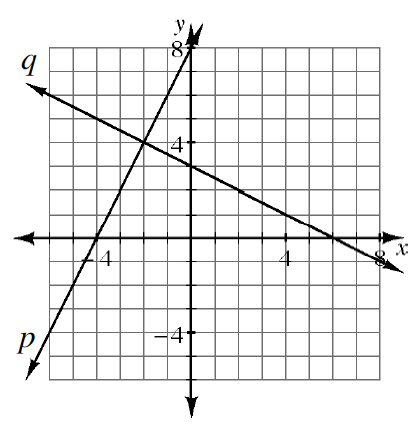### Home > CCG > Chapter 2 > Lesson 2.3.1 > Problem2-105

2-105.

One of the algebra topics you have reviewed during this chapter is solving systems of equations. Assess what you know about solving systems as you answer the questions below.

1. Find the points of intersection of the lines below using any method. Write your solutions as a point $(x,y)$.

(1) $\begin{array}[t]{l} y=-x+8\\ y=x-2 \end{array}$

(2) $\begin{array}[t]{l} 2x-y=10\\ y=-4x+2 \end{array}$

One way is to graph the two lines and find the point where the lines intersect.

If you use substitution, be careful when distributing the negative.

$\begin{array}{r} 2x-(-4x+2)=10\\ 2x+4x-2=10\\ 6x=12 \end{array}$

Solve for $y$.

2. Find the equation for each line on the graph at right. Remember, the general form of any line in the slope-intercept form is $y=mx+b$.Find the slope ($m$) by calculating the change in $y$ over the change in $x$, and then find the $y$-intercept ($b$) by looking at the graph.

1. What is the relationship between the two lines in part (b)? How do you know?

Use either substitution or elimination to solve the system.

2. Solve the system of equations you found in part (b) algebraically. Verify that your solution matches the one shown in the graph above.